# watershed

Watershed transform

## Syntax

``L = watershed(A)``
``L = watershed(A,conn)``

## Description

The watershed transform finds "catchment basins" or "watershed ridge lines" in an image by treating it as a surface where light pixels represent high elevations and dark pixels represent low elevations. The watershed transform can be used to segment contiguous regions of interest into distinct objects.

example

````L = watershed(A)` returns a label matrix `L` that identifies the watershed regions of the input matrix `A`.```
````L = watershed(A,conn)` specifies the connectivity to be used in the watershed computation.```

## Examples

collapse all

Create a binary image containing two overlapping circular objects. Display the image.

```center1 = -40; center2 = -center1; dist = sqrt(2*(2*center1)^2); radius = dist/2 * 1.4; lims = [floor(center1-1.2*radius) ceil(center2+1.2*radius)]; [x,y] = meshgrid(lims(1):lims(2)); bw1 = sqrt((x-center1).^2 + (y-center1).^2) <= radius; bw2 = sqrt((x-center2).^2 + (y-center2).^2) <= radius; bw = bw1 | bw2; imshow(bw) title('Binary Image with Overlapping Objects')```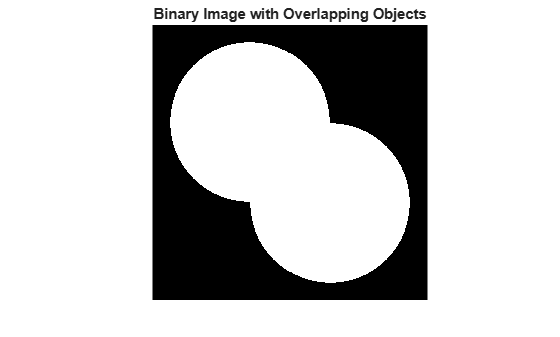Calculate the distance transform of the complement of the binary image. The value of each pixel in the output image is the distance between that pixel and the nearest nonzero pixel of `bw`.

```D = bwdist(~bw); imshow(D,[]) title('Distance Transform of Binary Image')```Take the complement of the distance transformed image so that light pixels represent high elevations and dark pixels represent low elevations for the watershed transform.

```D = -D; imshow(D,[]) title('Complement of Distance Transform')```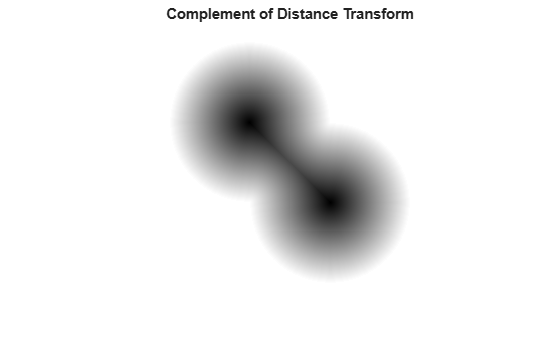Calculate the watershed transform. Set pixels that are outside the ROI to `0`.

```L = watershed(D); L(~bw) = 0;```

Display the resulting label matrix as an RGB image.

```rgb = label2rgb(L,'jet',[.5 .5 .5]); imshow(rgb) title('Watershed Transform')```Make a 3-D binary image containing two overlapping spheres.

```center1 = -10; center2 = -center1; dist = sqrt(3*(2*center1)^2); radius = dist/2 * 1.4; lims = [floor(center1-1.2*radius) ceil(center2+1.2*radius)]; [x,y,z] = meshgrid(lims(1):lims(2)); bw1 = sqrt((x-center1).^2 + (y-center1).^2 + ... (z-center1).^2) <= radius; bw2 = sqrt((x-center2).^2 + (y-center2).^2 + ... (z-center2).^2) <= radius; bw = bw1 | bw2; figure, isosurface(x,y,z,bw,0.5), axis equal, title('BW') xlabel x, ylabel y, zlabel z xlim(lims), ylim(lims), zlim(lims) view(3), camlight, lighting gouraud```Compute the distance transform.

```D = bwdist(~bw); figure, isosurface(x,y,z,D,radius/2), axis equal title('Isosurface of distance transform') xlabel x, ylabel y, zlabel z xlim(lims), ylim(lims), zlim(lims) view(3), camlight, lighting gouraud```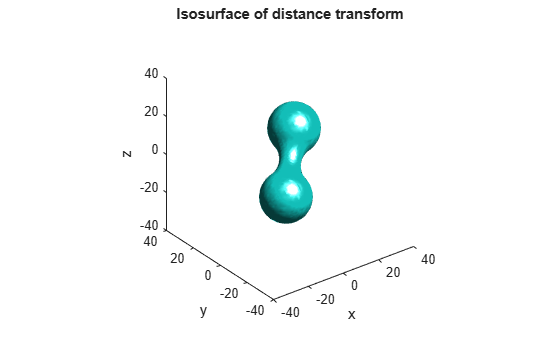Complement the distance transform, force nonobject pixels to be `Inf`, and then compute the watershed transform.

```D = -D; D(~bw) = Inf; L = watershed(D); L(~bw) = 0; figure isosurface(x,y,z,L==1,0.5) isosurface(x,y,z,L==2,0.5) axis equal title('Segmented objects') xlabel x, ylabel y, zlabel z xlim(lims), ylim(lims), zlim(lims) view(3), camlight, lighting gouraud```## Input Arguments

collapse all

Input image, specified as a numeric or logical array of any dimension.

Data Types: `single` | `double` | `int8` | `int16` | `int32` | `int64` | `uint8` | `uint16` | `uint32` | `uint64` | `logical`

Pixel connectivity, specified as one of the values in this table. The default connectivity is `8` for 2-D images, and `26` for 3-D images.

Value

Meaning

Two-Dimensional Connectivities

`4`

Pixels are connected if their edges touch. The neighborhood of a pixel are the adjacent pixels in the horizontal or vertical direction.Current pixel is shown in gray.

`8`

Pixels are connected if their edges or corners touch. The neighborhood of a pixel are the adjacent pixels in the horizontal, vertical, or diagonal direction.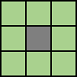Current pixel is shown in gray.

Three-Dimensional Connectivities

`6`

Pixels are connected if their faces touch. The neighborhood of a pixel are the adjacent pixels in:

• One of these directions: in, out, left, right, up, and downCurrent pixel is shown in gray.

`18`

Pixels are connected if their faces or edges touch. The neighborhood of a pixel are the adjacent pixels in:

• One of these directions: in, out, left, right, up, and down

• A combination of two directions, such as right-down or in-upCurrent pixel is center of cube.

`26`

Pixels are connected if their faces, edges, or corners touch. The neighborhood of a pixel are the adjacent pixels in:

• One of these directions: in, out, left, right, up, and down

• A combination of two directions, such as right-down or in-up

• A combination of three directions, such as in-right-up or in-left-down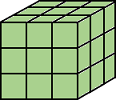Current pixel is center of cube.

For higher dimensions, `watershed` uses the default value `conndef(ndims(A),'maximal')`.

Connectivity can also be defined in a more general way for any dimension by specifying a 3-by-3-by- ... -by-3 matrix of `0`s and `1`s. The `1`-valued elements define neighborhood locations relative to the center element of `conn`. Note that `conn` must be symmetric about its center element. See Specifying Custom Connectivities for more information.

Note

If you specify a nondefault connectivity, pixels on the edge of the image might not be considered to be border pixels. For example, if `conn = [0 0 0; 1 1 1; 0 0 0]`, elements on the first and last row are not considered to be border pixels because, according to that connectivity definition, they are not connected to the region outside the image.

Data Types: `double` | `logical`

## Output Arguments

collapse all

Label matrix, specified as a numeric array of nonnegative integers. The elements labeled `0` do not belong to a unique watershed region. The elements labeled `1` belong to the first watershed region, the elements labeled 2 belong to the second watershed region, and so on.

## Tips

• The watershed transform algorithm used by this function changed in version 5.4 (R2007a) of the Image Processing Toolbox™ software. The previous algorithm occasionally produced labeled watershed basins that were not contiguous. If you need to obtain the same results as the previous algorithm, use the function `watershed_old`.

• To prevent oversegmentation, remove shallow minima from the image by using the `imhmin` function before you use the `watershed` function.

## Algorithms

`watershed` uses the Fernand Meyer algorithm .

 Meyer, Fernand, "Topographic distance and watershed lines,” Signal Processing , Vol. 38, July 1994, pp. 113-125.